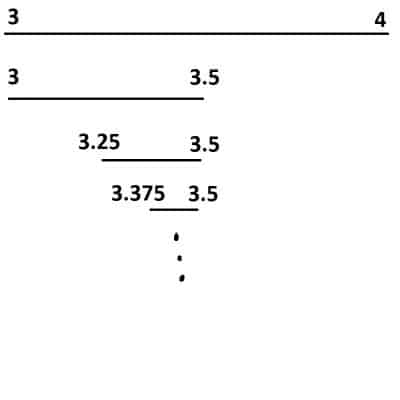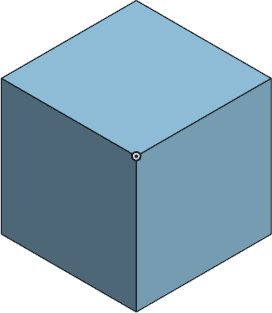# How To Do Square Roots By Hand (2 Key Steps)

Usually we can recognize small perfect squares and find their square roots without too much trouble.  However, it is a little more work to find the square root of a number that is not a perfect square.

So, how can you do square roots by hand?  To find √N by hand, start by squaring integers (12 = 1, 22 = 4, 32 = 9, etc.). Find the first number S such that S2 > N. Then S-1 < √N < S. Take the average of S-1 and S, and then square it. If the result is larger than N, try a smaller number; otherwise, try a larger number, and repeat the process.

Of course, you have to stop this process at some point.  However, you will be able to get an answer within 0.01 of √N in 7 steps, and within 0.001 of √N in 10 steps.

In this article, we’ll talk about how to find a square root by hand, giving the steps in detail.  We’ll also take a look at some examples.

Let’s get started.

## How To Do Square Roots By Hand

To find the square root of a number N by hand, there are two key steps:

• 1. First, we “bound” √N between two whole numbers, S-1 and S.  We do this by squaring integers (12 = 1, 22 = 4, 32 = 9, etc.) until we find a result S2 that is larger than N.
• 2. Second, we repeatedly “cut in half” the boundary interval for √N by taking the average of the endpoints and squaring the result.  If we get something larger than N, we take a smaller number.  If we get something smaller than N, we take a larger number.

It will help to see some examples of how this works, so let’s start with a few.When we find the square root of a number N, we are finding the side length of a square with an area of N.

### Example 1: How To Find The Square Root Of 12 By Hand

To find √12 by hand, the first step is to list out perfect squares until we find one larger than N = 12:

• 12 = 1
• 22 = 4
• 32 = 9
• 42 = 16

We stop at S = 4, since 42 = 16 is the first squared integer larger than N = 12.

This means that our boundary is S-1 < √N < S, or 3 < √12 < 4.

In step 2, we find a more exact number for √12 by repeating the following process.

First, take the average of the endpoints: (3 + 4) / 2 = 3.5.

Next, square the average we found: 3.52 = 12.25.

Now, since the result of 12.25 is larger than 12, we want a smaller number.

Our boundary is now 3 < √12 < 3.5.

Repeating the process:

First, take the average of the endpoints: (3 + 3.5) / 2 = 3.25.

Next, square the average we found: 3.252 = 10.5625.

Now, since the result of 10.5625 is smaller than 12, we want a larger number.

Our boundary is now 3.25 < √12 < 3.5.

Repeating the process:

First, take the average of the endpoints: (3.25 + 3.5) / 2 = 3.375.

Next, square the average we found: 3.3752 = 11.390625.

Now, since the result of 11.390625 is smaller than 12, we want a larger number.

Our boundary is now 3.375 < √12 < 3.5.

Repeating the process:

First, take the average of the endpoints: (3.375 + 3.5) / 2 = 3.4375.

Next, square the average we found: 3.43752 = 11.81640625.

Now, since the result of 11.81640625 is smaller than 12, we want a larger number.

Our boundary is now 3.4375 < √12 < 3.5.

Repeating the process:

First, take the average of the endpoints: (3.4375 + 3.5) / 2 = 3.46875.

Next, square the average we found: 3.468752 = 12.0322265625.

Now, since the result of 12.0322265625 is larger than 12, we want a smaller number.

Our boundary is now 3.4375 < √12 < 3.46875.

Repeating the process:

First, take the average of the endpoints: (3.4375 + 3.46875) / 2 = 3.453125.

Next, square the average we found: 3.4531252 = 11.924072265625.

Now, since the result of 11.924072265625 is smaller than 12, we want a larger number.

Our boundary is now 3.453125 < √12 < 3.46875.

At this point, the boundary is now only about 0.015 wide.  If we take the average of the two endpoints now, we will get a value that is within about 0.0075 of √12.

So, we take 3.4609375 as our value.  When squared, this gives us about 11.978, which is within 0.022 of 12, or an error of about 0.022/12 = 0.2%.To take the square root of 12, we bound the value between 3 and 4 (whole numbers). Then, we cut the interval in half repeatedly, getting closer to the square root of 12 at each step.

### Example 2: How To Find The Square Root Of 28 By Hand

Now that we are familiar with the process, we will try finding another square root, √28.

We know that 52 = 25 and 62 = 36, so √28 is between 5 and 6.  Taking averages and squaring:

• 5.52 = 30.25 (too large, so √28 is between 5 and 5.5)
• 5.252 = 27.5625 (too small, so √28 is between 5.25 and 5.5)
• 5.3752 = 28.890625 (too large, so √28 is between 5.25 and 5.375)
• 5.31252 = 28.22265625 (too large, so √28 is between 5.25 and 5.3125)
• 5.281252 = 27.8916015625 (too small, so √28 is between 5.28125 and 5.3125)
• 5.2968752 = 28.056884765625 (too large, so √28 is between 5.28125 and 5.296875)

Taking the average of the endpoints of the boundary, we get a value of 5.2890625.  When we square it, we get about 27.974, which is within about 0.026 of 28, or an error of 0.026/28 = 0.09%

## Calculating Cube Roots By Hand

The process for calculating cube roots by hand is similar.  As our first step, we still want to bound the cube root between two whole numbers.

Then, we want to continue making the boundary smaller until we get close to the cube root.When we find the cube root of a number N, we are finding the side length of a cube with a volume of N.

Let’s try an example to see how it works.

### Example: How To Find The Cube Root Of 15 By Hand

Now we will try finding the cube root of 15 by hand, or 3√15.

We know that 23 = 8 and 33 = 27, so 3√15 is between 2 and 3.  Taking averages and cubing:

• 2.53 = 15.625 (too large, so 3√15 is between 2 and 2.5)
• 2.253 = 11.390625 (too small, so 3√15 is between 2.25 and 2.5)
• 2.3753 = 13.396484375 (too small, so 3√15 is between 2.375 and 2.5)
• 2.43753 = 14.482177734375 (too small, so 3√15 is between 2.4375 and 2.5)
• 2.468753 = 15.04635620117175 (too large, so 3√15 is between 2.4375 and 2.46875)

We will take 2.46875 as our cube root of 15.  This is within ½5 or 1/32 (about 3%) of 3√15.  The cubed number is within about 0.046 of 15, or an error of 0.046/15 = 0.3%.

## Conclusion

Now you know how to do square roots by hand (and also cube roots).  You can get as close as you want to the square root of a number with this method, but the calculations can become tedious as you get longer decimal expansions.

You can learn how to add, multiply, and divide square roots here.